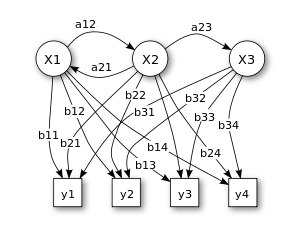## Hidden Markov ModelHidden Markov Model (HMM) is a statistical Markov model in which the system being modeled is assumed to be a Markov process – call it X – with unobservable ("hidden") states.

Machine learningProbabilistic Graphical Model

true

#### Classification(s)

Method-focused categoriesData-perspectiveIntelligent computation analysis

#### Detailed Description

English {{currentDetailLanguage}} English

The diagram below shows the general architecture of an instantiated HMM. Each oval shape represents a random variable that can adopt any of a number of values. The random variable x(t) is the hidden state at time t (with the model from the above diagram, x(t) ∈ { x1x2x3 }). The random variable y(t) is the observation at time t (with y(t) ∈ { y1y2y3y4 }). The arrows in the diagram (often called a trellis diagram) denote conditional dependencies.

From the diagram, it is clear that the conditional probability distribution of the hidden variable x(t) at time t, given the values of the hidden variable x at all times, depends only on the value of the hidden variable x(t − 1); the values at time t − 2 and before have no influence. This is called the Markov property. Similarly, the value of the observed variable y(t) only depends on the value of the hidden variable x(t) (both at time t).

In the standard type of hidden Markov model considered here, the state space of the hidden variables is discrete, while the observations themselves can either be discrete (typically generated from a categorical distribution) or continuous (typically from a Gaussian distribution). The parameters of a hidden Markov model are of two types, transition probabilities and emission probabilities (also known as output probabilities). The transition probabilities control the way the hidden state at time t is chosen given the hidden state at time.

The hidden state space is assumed to consist of one of N possible values, modelled as a categorical distribution. (See the section below on extensions for other possibilities.) This means that for each of the N possible states that a hidden variable at time t can be in, there is a transition probability from this state to each of the N possible states of the hidden variable at time, for a total oftransition probabilities. Note that the set of transition probabilities for transitions from any given state must sum to 1. Thus, thematrix of transition probabilities is a Markov matrix. Because any one transition probability can be determined once the others are known, there are a total oftransition parameters.

In addition, for each of the N possible states, there is a set of emission probabilities governing the distribution of the observed variable at a particular time given the state of the hidden variable at that time. The size of this set depends on the nature of the observed variable. For example, if the observed variable is discrete with M possible values, governed by a categorical distribution, there will beseparate parameters, for a total ofemission parameters over all hidden states. On the other hand, if the observed variable is an M-dimensional vector distributed according to an arbitrary multivariate Gaussian distribution, there will be M parameters controlling the means andparameters controlling the covariance matrix, for a total ofemission parameters. (In such a case, unless the value of M is small, it may be more practical to restrict the nature of the covariances between individual elements of the observation vector, e.g. by assuming that the elements are independent of each other, or less restrictively, are independent of all but a fixed number of adjacent elements.)

#### {{htmlJSON.HowtoCite}}

Zhen Qian (2020). Hidden Markov Model, Model Item, OpenGMS, https://geomodeling.njnu.edu.cn/modelItem/3c01e4fa-4e74-45ae-aea3-630a8199d64e

#### QR Code{{curRelation.name}}
• {{curRelation.name}}
{{curRelation.name}}

{{curRelation.overview}}
{{curRelation.author.join('; ')}}
{{curRelation.journal}}

{{htmlJSON.RelatedItems}}
{{props.row.name}}
••••{{ props.row.overview }}
{{ props.row.overview }}
Drop the file here, orclick to upload.
File size should not exceed 10m.
Select From My Space

{{htmlJSON.authorshipSubmitted}}

Cancel Submit
{{htmlJSON.Cancel}} {{htmlJSON.Submit}}
{{ item.label }} {{ item.value }}
{{props.row.localName}}
{{htmlJSON.ModelName}}:
{{htmlJSON.Cancel}} {{htmlJSON.Submit}}
模型名称
名称 别名 {{tag}}

模型版本
系列名 版本号 目的 修改内容 创建/修改日期 作者

描述信息
摘要 详细描述

{{tag}}
* 时间参考系
* 空间参考系类型 * 空间参考系名称

开发信息
起始日期 终止日期 进展 开发者

* 是否开源 * 访问方式 * 使用方式 开源协议 * 传输方式 * 获取地址 * 发布日期 * 发布者

元数据版本
编号 目的 修改内容 创建/修改日期 作者
{{index+1}}

{{index+1}}

{{index+1}}

模型类型

分类信息

时间分辨率 时间尺度 时间步长 时间范围 空间维度 格网类型 空间分辨率 空间尺度 空间范围
{{tag}}
* 类型
图例

* 名称 * 描述
上传

示例描述 * 名称 * 类型 * 值/链接 上传

{{htmlJSON.Cancel}} {{htmlJSON.Submit}}
Title Author Date Journal Volume(Issue) Pages Links Doi Operation
{{htmlJSON.Cancel}} {{htmlJSON.Submit}}

Yes, this is it Cancel

OK
{{htmlJSON.Cancel}} {{htmlJSON.Confirm}}
Model Classifications 1
Model Classifications 2Title Author Date Journal Volume(Issue) Pages Links Doi Operation

#### NEW

Name:
Affiliation:
Email:
Homepage:

Yes, this is it Cancel

Confirm
{{htmlJson.path}}
:
/{{path.name}}
search results of '{{searchContentShown}}'

#### No content to show

{{item.name}}

.

{{item.suffix}}

.{{item.suffix}}

{{htmlJson.Max}}: {{toDecimal1(capacity/1073741824)}} GB
Copy
Delete
Rename
/{{path.label}}
{{htmlJson.Change}}
/{{path.name}}
{{htmlJson.SelectFile}}
{{htmlJson.Cancel}} {{htmlJson.Confirm}}
{{htmlJson.path}}
:
/{{path.name}}
/..
{{htmlJson.Cancel}} {{htmlJson.Confirm}}
{{ data.name }}
##### You have select  {{multipleSelection.length+multipleSelectionMyData.length}} data .
• Output Data
• {{item.computableName}}@{{formatDate(item.runTime)}}
{{scope.row.type}}
{{ scope.row.tag }}
• Fork Data
{{it.category}}

#### NEW

Name:
Affiliation:
Email:
Homepage:
previous next conform
{{htmlJSON.ModelClassifications}}

{{htmlJson.RelatedItems}}
{{ props.row.overview }}
{{ props.row.overview }}
{{htmlJson.Cancel}} {{htmlJson.OK}}
{{ item.label }} {{ item.value }}
{{props.row.localName}}
Model Name :